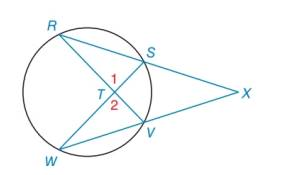Chapter 6.2, Problem 33E### Elementary Geometry for College St...

6th Edition
Daniel C. Alexander + 1 other
ISBN: 9781285195698

#### Solutions

Chapter
Section### Elementary Geometry for College St...

6th Edition
Daniel C. Alexander + 1 other
ISBN: 9781285195698
Textbook Problem
1 views

# In the figure shown, Δ R X V ∼ Δ W X S by the reason A A . Name two pairs of congruent angles in these similar triangles.Exercises 32, 33

To determine

To name:

The two pairs of congruent angles in the given similar triangles.

Explanation

Calculation:

Given, ΔRXVΔWXS by the reason of AA similarity.

Consider the figure,

By the vertical angle theorem,

1=2.

Therefore, from the figure, RW

### Still sussing out bartleby?

Check out a sample textbook solution.

See a sample solution

#### The Solution to Your Study Problems

Bartleby provides explanations to thousands of textbook problems written by our experts, many with advanced degrees!

Get Started

#### Find more solutions based on key concepts